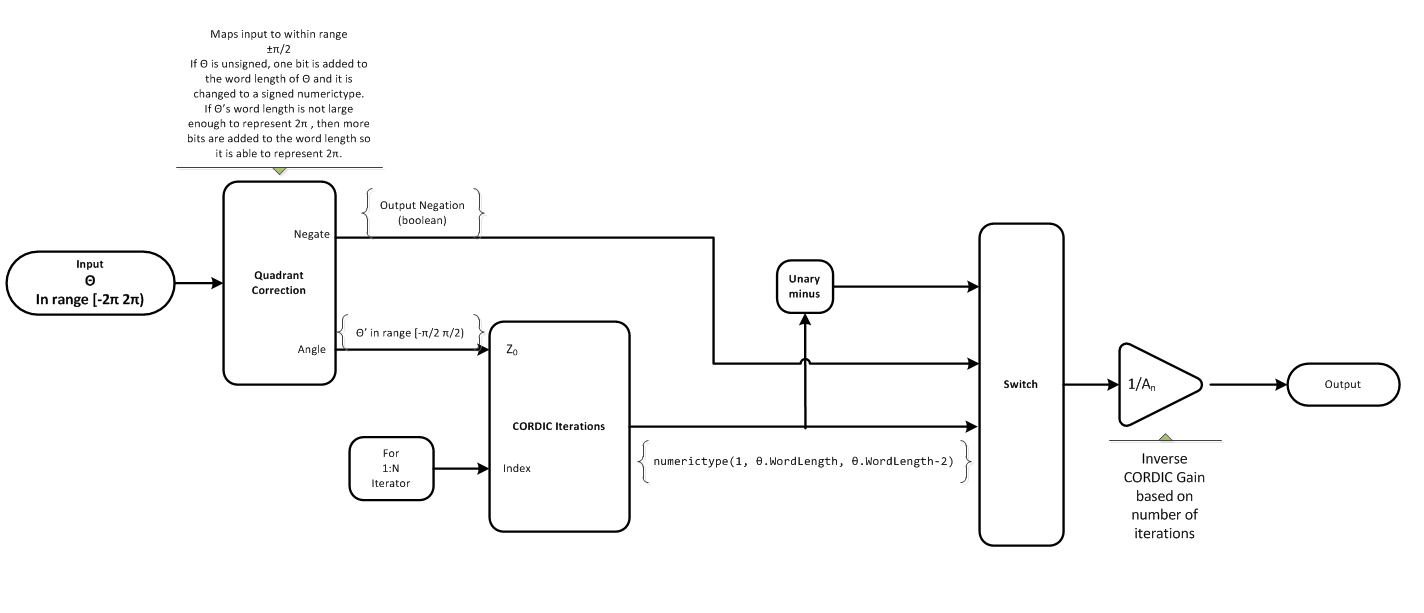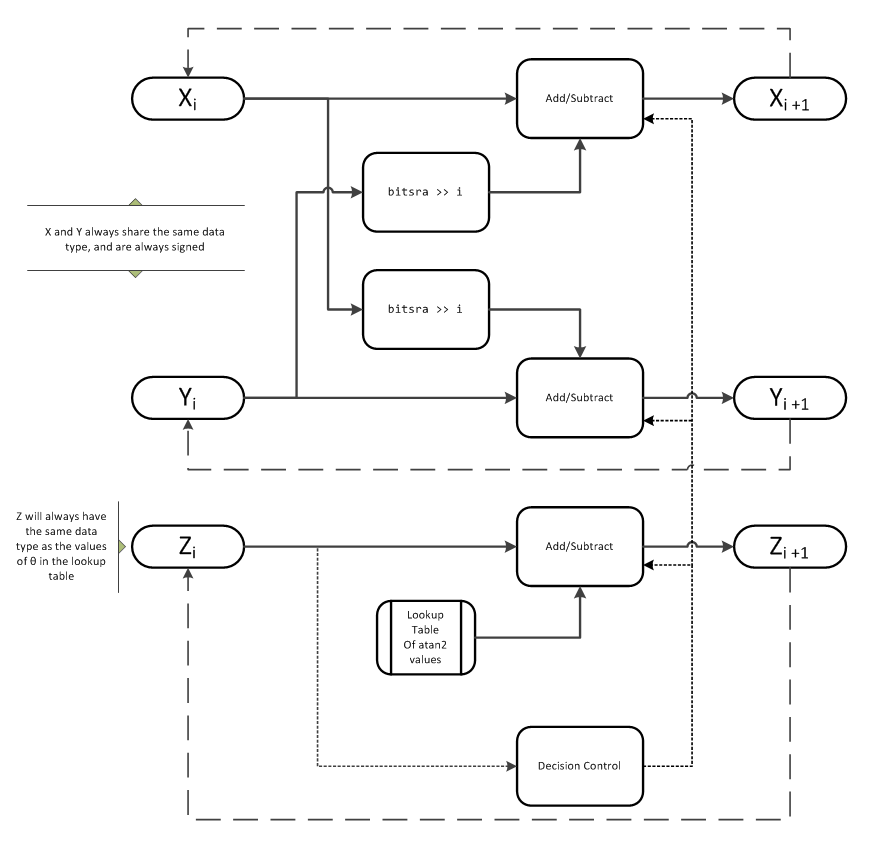# cordiccexp

CORDIC-based approximation of complex exponential

## Syntax

```y = cordiccexp(theta,niters) ```

## Description

`y = cordiccexp(theta,niters)` computes `cos`(`theta`) + j*`sin`(`theta`) using a CORDIC algorithm approximation. `y` contains the approximated complex result.

## Input Arguments

 `theta` `theta` can be a signed or unsigned scalar, vector, matrix, or `N`-dimensional array containing the angle values in radians. All values of `theta` must be real and in the range [–2π 2π). `niters` `niters` is the number of iterations the CORDIC algorithm performs. This is an optional argument. When specified, `niters` must be a positive, integer-valued scalar. If you do not specify `niters` or if you specify a value that is too large, the algorithm uses a maximum value. For fixed-point operation, the maximum number of iterations is one less than the word length of `theta`. For floating-point operation, the maximum value is 52 for double or 23 for single. Increasing the number of iterations can produce more accurate results, but it also increases the expense of the computation and adds latency.

## Output Arguments

 `y` `y` is the approximated complex result of the `cordiccexp` function. When the input to the function is floating point, the output data type is the same as the input data type. When the input is fixed point, the output has the same word length as the input, and a fraction length equal to the `WordLength` – `2`.

## Examples

The following example illustrates the effect of the number of iterations on the result of the `cordiccexp` approximation.

```wrdLn = 8; theta = fi(pi/2, 1, wrdLn); fprintf('\n\nNITERS\t\tY (SIN)\t ERROR\t LSBs\t\tX (COS)\t ERROR\t LSBs\n'); fprintf('------\t\t-------\t ------\t ----\t\t-------\t ------\t ----\n'); for niters = 1:(wrdLn - 1) cis = cordiccexp(theta, niters); fl = cis.FractionLength; x = real(cis); y = imag(cis); x_dbl = double(x); x_err = abs(x_dbl - cos(double(theta))); y_dbl = double(y); y_err = abs(y_dbl - sin(double(theta))); fprintf('%d\t\t%1.4f\t %1.4f\t %1.1f\t\t%1.4f\t %1.4f\t %1.1f\n', niters, y_dbl, y_err,(y_err * pow2(fl)), x_dbl, x_err,(x_err * pow2(fl))); end fprintf('\n'); ```

The output table appears as follows:

```NITERS Y (SIN) ERROR LSBs X (COS) ERROR LSBs ------ ------- ------ ---- ------- ------ ---- 1 0.7031 0.2968 19.0 0.7031 0.7105 45.5 2 0.9375 0.0625 4.0 0.3125 0.3198 20.5 3 0.9844 0.0156 1.0 0.0938 0.1011 6.5 4 0.9844 0.0156 1.0 -0.0156 0.0083 0.5 5 1.0000 0.0000 0.0 0.0312 0.0386 2.5 6 1.0000 0.0000 0.0 0.0000 0.0073 0.5 7 1.0000 0.0000 0.0 0.0156 0.0230 1.5```

collapse all

### CORDIC

CORDIC is an acronym for COordinate Rotation DIgital Computer. The Givens rotation-based CORDIC algorithm is one of the most hardware-efficient algorithms available because it requires only iterative shift-add operations (see References). The CORDIC algorithm eliminates the need for explicit multipliers. Using CORDIC, you can calculate various functions such as sine, cosine, arc sine, arc cosine, arc tangent, and vector magnitude. You can also use this algorithm for divide, square root, hyperbolic, and logarithmic functions.

Increasing the number of CORDIC iterations can produce more accurate results, but doing so increases the expense of the computation and adds latency.

## Algorithms

collapse all

### Signal Flow DiagramsCORDIC Rotation KernelX represents the real part, Y represents the imaginary part, and Z represents theta. The accuracy of the CORDIC rotation kernel depends on the choice of initial values for X, Y, and Z. This algorithm uses the following initial values:

### fimath Propagation Rules

CORDIC functions discard any local `fimath` attached to the input.

The CORDIC functions use their own internal `fimath` when performing calculations:

• `OverflowAction``Wrap`

• `RoundingMethod``Floor`

The output has no attached `fimath`.

## Version History

Introduced in R2010a